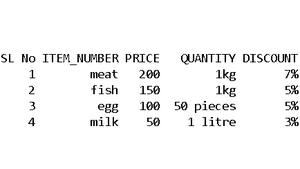# How to create Pandas DataFrame from nested XML?

In this article, we will learn how to create Pandas DataFrame from nested XML. We will use the xml.etree.ElementTree module, which is a built-in module in Python for parsing or reading information from the XML file. The ElementTree represents the XML document as a tree and the Element represents only a single node of the tree.

### Functions Used:

Here, we will use some functions to process out code which is stated below:

• ElementTree.parse( XML_file) : To read data from an XML file
• root.iter(‘root_name’): To iterate through the branches of the root node
• ElementTree.fromstring(XML_file) : To read data when XML code which is passed as a string inside triple quotes in the python code
• prstree.findall(‘store’): To find all the elements of the parsed XML ElementTree
• node.attribute.get(attribte_name ): To get the attribute
• node.find(attribte_name): To retrieve the text content of the mentioned attribute_name
• pandas.DataFrame() : To convert the XML data to a DataFrame
• list.append(): To append the items to a list

### Approach

• Parse or read the XML file using ElementTree.parse( ) function and get the root element.
• Iterate through the root node to get the child nodes attributes ‘SL NO’ (here) and extract the text values of each attribute (here foodItem, price, quantity, and discount).
• Get the respective food items with specifications as a unit appended to a list(here all_items() list).
• Convert the list into a DataFrame using pandas.DataFrame() function and mention the column names within quotes separated by commas.
• Print the DataFrame and it’s done.

## XML

 ` ` ` `  `       ``<``Food``> ` ` `  `           ``<``Info``> ` `           ``<``Msg``>Food Store items. ` `           `` ` ` `  `           ``<``store` `slNo``=``"1"``> ` `               ``<``foodItem``>meat ` `               ``<``price``>200 ` `               ``<``quantity``>1kg ` `               ``<``discount``>7% ` `           `` ` ` `  `           ``<``store` `slNo``=``"2"``> ` `               ``<``foodItem``>fish ` `               ``<``price``>150 ` `               ``<``quantity``>1kg ` `               ``<``discount``>5% ` `           `` ` ` `  `           ``<``store` `slNo``=``"3"``> ` `               ``<``foodItem``>egg ` `               ``<``price``>100 ` `               ``<``quantity``>50 pieces ` `               ``<``discount``>5% ` `           `` ` ` `  `           ``<``store` `slNo``=``"4"``> ` `               ``<``foodItem``>milk ` `               ``<``price``>50 ` `               ``<``quantity``>1 litre ` `               ``<``discount``>3% ` `           `` ` ` `  `       ```

Example 1:

In this code below we have parsed the XML file. Give the complete path where you have saved the XML file within quotes. So here we need to use ElementTree.parse() function to read the data from the XML file and then the getroot() function to get the root. Then follow the steps given.

## Python3

 `import` `xml.etree.ElementTree as ETree ` `import` `pandas as pd ` ` `  `# give the path where you saved the xml file ` `# inside the quotes ` `xmldata ``=` `"C: \\ProgramData\\Microsoft\\ ` `    ``Windows\\Start Menu\\Programs\\ ` `    ``Anaconda3(``64``-``bit)\\xmltopandas.xml" ` `prstree ``=` `ETree.parse(xmldata) ` `root ``=` `prstree.getroot() ` ` `  `# print(root) ` `store_items ``=` `[] ` `all_items ``=` `[] ` ` `  `for` `storeno ``in` `root.``iter``(``'store'``): ` `   `  `    ``store_Nr ``=` `storeno.attrib.get(``'slNo'``) ` `    ``itemsF ``=` `storeno.find(``'foodItem'``).text ` `    ``price ``=` `storeno.find(``'price'``).text ` `    ``quan ``=` `storeno.find(``'quantity'``).text ` `    ``dis ``=` `storeno.find(``'discount'``).text ` ` `  `    ``store_items ``=` `[store_Nr, itemsF, price, quan, dis] ` `    ``all_items.append(store_items) ` ` `  `xmlToDf ``=` `pd.DataFrame(all_items, columns``=``[ ` `  ``'SL No'``, ``'ITEM_NUMBER'``, ``'PRICE'``, ``'QUANTITY'``, ``'DISCOUNT'``]) ` ` `  `print``(xmlToDf.to_string(index``=``False``)) `

Output:Note: The XML file should be saved in the same directory or folder where your Python code saved.

Example 2:

We can also pass the XML content as a string inside triple quotes. In that case, we need to use the fromstring() function to read the string. Get the root using the ‘tag’ object and follow the same steps to convert it to a DataFrame as mentioned above.

## Python3

 `import` `xml.etree.ElementTree as ETree ` `import` `pandas as pd ` ` `  `xmldata ``=` `''' ` `        `` ` `            `` ` `            ``Food Store items. ` `            `` ` `            `` ` `                ``meat ` `                ``200 ` `                ``1kg ` `                ``7% ` `            `` ` `            `` ` `                ``fish ` `                ``150 ` `                ``1kg ` `                ``5% ` `            `` ` `            `` ` `                ``egg ` `                ``100 ` `                ``50 pieces ` `                ``5% ` `            `` ` `            `` ` `                ``milk ` `                ``50 ` `                ``1 litre ` `                ``3% ` `            `` ` `        `` ` `'''` ` `  `prstree ``=` `ETree.fromstring(xmldata) ` `root ``=` `prstree.tag ` ` `  `#print(root) ` `store_items ``=` `[] ` `all_items ``=` `[] ` ` `  `for` `storeno ``in` `prstree.findall(``'store'``):   ` `    ``store_Nr ``=` `storeno.attrib.get(``'slNo'``) ` `    ``itemsF``=` `storeno.find(``'foodItem'``).text ` `    ``price``=` `storeno.find(``'price'``).text ` `    ``quan``=` `storeno.find(``'quantity'``).text ` `    ``dis``=` `storeno.find(``'discount'``).text ` `         `  `    ``store_items ``=` `[store_Nr,itemsF,price,quan,dis] ` `    ``all_items.append(store_items) ` ` `  `xmlToDf ``=` `pd.DataFrame(all_items,columns``=``[ ` `  ``'SL No'``,``'ITEM_NUMBER'``,``'PRICE'``,``'QUANTITY'``,``'DISCOUNT'``])         ` ` `  `print``(xmlToDf.to_string(index``=``False``))`

Output:Whether you're preparing for your first job interview or aiming to upskill in this ever-evolving tech landscape, GeeksforGeeks Courses are your key to success. We provide top-quality content at affordable prices, all geared towards accelerating your growth in a time-bound manner. Join the millions we've already empowered, and we're here to do the same for you. Don't miss out - check it out now!

Previous
Next